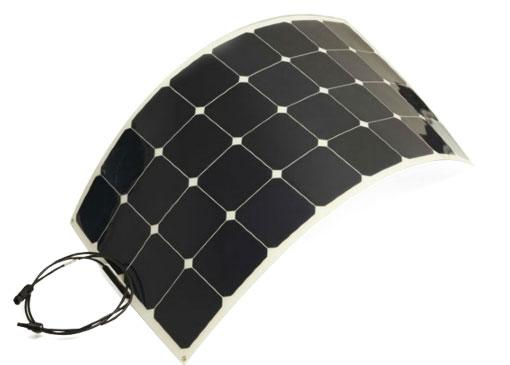..one stop store for your needs delivering trust
TUTORIAL: OFF GRID SOLAR SYSTEM DESIGN

This tutorial would help you in understanding and implementing small scale solar system design project, ideally suited for homesSteps to be followed
1 Conduct Energy Audit of the place where the solar system is to be installed, e.g, your house. Assess the energy required by various appliances in your house..Your Electricity bills will give you the idea of the energy requirement for different parts in the year
 Appliance Name Number of the appliance Power required by the appliance Time for which device in ON in a day Energy requirement per day Total Energy requirement per day(A)
2

Decide on battery system voltage to be used. Typically for small loads (<1 KWh), 12 V systems are used, for loads >1 KWh  but < 4 KWh, 24 V systems  and for larger loads 48V systems are used. If battery & Inverter are at large distances from energy source, then higher voltage systems are preferred to minimise IR losses

System Voltage(B) = .....................

3

Select Battery: Choice of battery is governed by various factors. So, follow the steps given

3a

To size the battery, divide total energy required by the system voltage.

Therfore, Battery capacity = Total energy / system voltage

3b

Redjust Battery Capacity based on days of Autonomy.
Therefore , required battery capacity = Battery capacity * Days of Autonomy

The battery capacity should be greater of the two: - Energy demand for days of autonomy & Peak power demand

3c Obtain the details of available battery with the chosen system Voltage and battery capacity closer to the battery capacity computed in step 3b. Deep cycle batteries should be preferred.
3d

Battery have recommended depth of discharge(DOD). Obtain recommended depth of discharge for the battery of choise.

Accordingly, battery capacity should be re-adjusted. If max. DOD is exceeded, battery life is effected

Readjusted Battery capacity = Battery capacity / DOD

3d

Redjust Battery Capacity based on Battery Discharge Rate (C)
Some appliance draw power only for small periods, which affects the battery
When power usage rate is not high, battery is selected at 100 hr rate of discharge. If power usage rates are high, battery is selected at 10 hr/20 hr  rate of discharge

Choose Deep discharge batteries for the required system voltage & capacity. parallel strings of battery are not recommended. If necessary, use each string with a fuse

Final Battery Capacity(C) = .....................

You may need to repeat steps 3c & 3d to zero-down on the appropriate battery.

4 To size PV array, follow the steps given below
4a Obtain Solar irradiation data for the location from meteorological department. Solar irradiation is expressed in kWh/m2 which can be translated to Peak Number of hours(PSH) of solar irradiance of 1kW/m2.
4b

Compute total energy required taking into account battery columbic efficiency

Total energy required(in Wh) = Energy requirement computed in step 1(in Wh)/ / battery efficiency

Total energy required(in Ah) = Energy requirement computed in step 1(in Wh)/(System Voltage* battery efficiency)

Now taking worst value of PSH ,compute required PV array output current.
PV array output current(D) = Total energy required(in Ah)/ PSH = .....................

4c

Include PV Derating factor
a) Check Mfr Power tolerance from datasheet of PV panel
b) Take 5% derating factor for dirt
c) Temperature  Modules’ output power decreases with temperature above 25°C and increases with temperatures below 25°C. The average cell temperature is taken as 25 deg higher than the ambient temperature.

Since the modules are used for battery charging, the current at 14 Volts at the effective cell temperature should be used in calculations. In case curves are unavailable to determine the current at effective cell temperature then use the Normal Operating Cell temperature (NOCT) provided by the manufacturers.

Taking Derating factors into account, compute PV panel output current
Readjusted I(NOCT)  = I(NOCT)  X (1-Mfgr Tolerance) X 0.95 = (E).....................

4d

Now, you would need to select commercially available PV modules.

Vmp (at max. temperature of operation) of the module/array should be higher than the system voltage .If nominal operating voltage of each module is less than that of system voltage, PV modules need to be connected in series.

To compute number of Modules in a ArrayNumber of Modules in a Array, Divide system voltage by nominal operating voltage of each module. This would give you the number of modules connected in series in a array.

No of modules in series= (F).....................

4e

If Isc of each module is less than Readjusted I(NOCT) , PV modules need to be connected in parallel.

Divide Readjusted I(NOCT) by PV Output Current by of each module. This would give you the number of modules connected in parallel in a array.

No of modules in parallel= (G).....................

5 Charge Controller
5a

Charge controller should be chosen in such a way that it is capable of carrying 125% of the array short circuit current and it can withstand the open circuit voltage of the array.

Current Rating of Charge Controller (H) > 1.25 times of Readjusted I(NOCT)

Voltage Rating of Charge Controller (I) > Voc of module * No of modules in series.

6 Inverter Selection
6a

The inverter should be capable of supplying continuous power to all AC loads AND providing sufficient surge capability to start any loads that may surge when turned on and particularly if they turn on at the same time.

Inverter selection must be done according to AC load requirement(computed in Step 1) and also taking Surge factor into account.

Inverter Rating=(H)..................

Relevant Products
Flexible Solar Panels